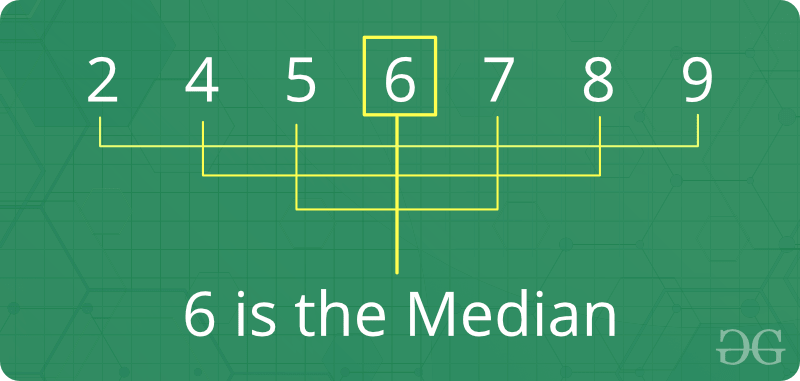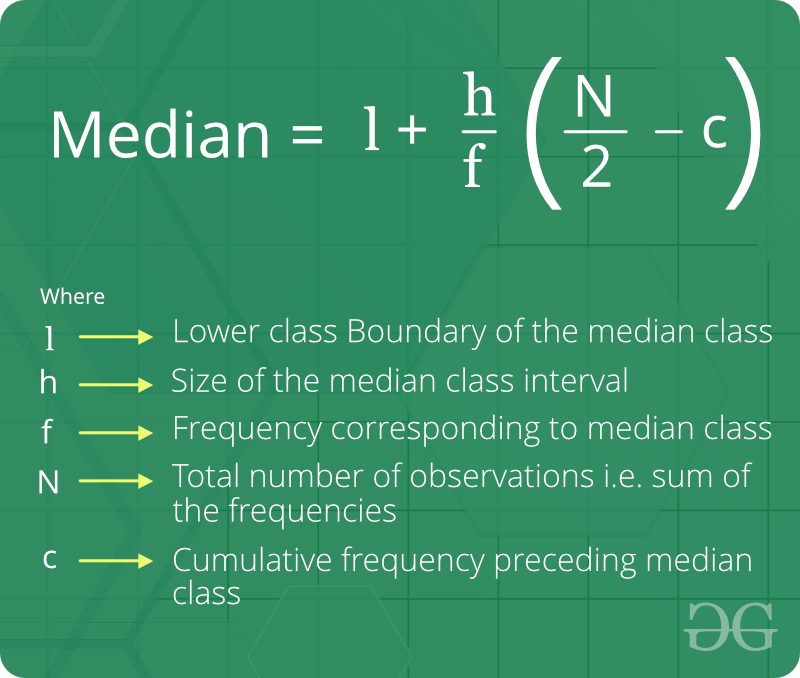Skip to content
Related Articles
Median
• Difficulty Level : Medium
• Last Updated : 07 Jun, 2021

Median is the middle value of a set of data. To determine the median value in a sequence of numbers, the numbers must first be arranged in ascending order.

• If there is an odd amount of numbers, the median value is the number that is in the middle, with the same amount of numbers below and above.
• If there is an even amount of numbers in the list, the median is the average of the two middle values.Fact about Median :

1. Median is joined by the mean and the mode to create a grouping called measures of central tendency.
2. Median is an important measure (compared to mean) for distorted data, because median is not so easily distorted. For example, median of {1, 2, 2, 5, 100) is 2 and mean is 22.
3. If user add a constant to every value, the mean and median increase by the same constant.
4. If user multiply every value by a constant, the mean and the median will also be multiplied by that constant.

Formula of Median of ungrouped data :Formula of Median of grouped data :How to find a median of an unsorted array ?
Naive solution:
Given n size unsorted array, find its median.

Median of a sorted array of size n is defined as below :
It is middle element when n is odd and average of middle two elements when n is even. Since the array is not sorted here, we sort the array first, then apply above formula.

Examples:

```Input  : {1, 3, 4, 2, 6, 5, 8, 7}
Output : Median = 4.5

Since number of elements are even, median
is average of 4th and 5th largest elements.
which means (4 + 5)/2 = 4.5

Input  : {4, 4, 4, 4, 4}
Output : Median = 4         ```

Below is the code implementation:

## C++

 `// CPP program to find median``#include ``using` `namespace` `std;` `// Function for calculating median``double` `findMedian(``int` `a[], ``int` `n)``{``    ``// First we sort the array``    ``sort(a, a + n);` `    ``// check for even case``    ``if` `(n % 2 != 0)``        ``return` `(``double``)a[n / 2];` `    ``return` `(``double``)(a[(n - 1) / 2] + a[n / 2]) / 2.0;``}` `// Driver program``int` `main()``{``    ``int` `a[] = { 1, 3, 4, 2, 7, 5, 8, 6 };``    ``int` `n = ``sizeof``(a) / ``sizeof``(a);``    ``cout << ``"Median = "` `<< findMedian(a, n) << endl;``    ``return` `0;``}`

## Java

 `// Java program to find median``import` `java.util.*;` `class` `GFG {` `    ``// Function for calculating median``    ``public` `static` `double` `findMedian(``int` `a[], ``int` `n)``    ``{``        ``// First we sort the array``        ``Arrays.sort(a);` `        ``// check for even case``        ``if` `(n % ``2` `!= ``0``)``            ``return` `(``double``)a[n / ``2``];` `        ``return` `(``double``)(a[(n - ``1``) / ``2``] + a[n / ``2``]) / ``2.0``;``    ``}` `    ``// Driver program``    ``public` `static` `void` `main(String args[])``    ``{``        ``int` `a[] = { ``1``, ``3``, ``4``, ``2``, ``7``, ``5``, ``8``, ``6` `};``        ``int` `n = a.length;``        ``System.out.println(``"Median = "` `+ findMedian(a, n));``    ``}``}`

## Python3

 `# Python3 program to find median` `# Function for calculating median``def` `findMedian(a, n):` `    ``# First we sort the array``    ``sorted``(a)` `    ``# check for even case``    ``if` `n ``%` `2` `!``=` `0``:``        ``return` `float``(a[n ``/``/` `2``])``    ` `    ``return` `float``((a[``int``((n``-``1``)``/``2``)] ``+``                  ``a[``int``(n ``/` `2``)])``/``2.0``)` `# Driver program``a ``=` `[ ``1``, ``3``, ``4``, ``2``, ``7``, ``5``, ``8``, ``6` `]``n ``=` `len``(a)``print``(``"Median ="``, findMedian(a, n))`

## C#

 `// C# program to find median``using` `System;` `class` `GFG {` `    ``// Function for``    ``// calculating median``    ``public` `static` `double` `findMedian(``int``[] a, ``int` `n)``    ``{``        ``// First we sort``        ``// the array``        ``Array.Sort(a);` `        ``// check for``        ``// even case``        ``if` `(n % 2 != 0)``            ``return` `(``double``)a[n / 2];` `        ``return` `(``double``)(a[(n - 1) / 2] + a[n / 2]) / 2.0;``    ``}` `    ``// Driver Code``    ``public` `static` `void` `Main()``    ``{``        ``int``[] a = { 1, 3, 4, 2,``                    ``7, 5, 8, 6 };``        ``int` `n = a.Length;``        ``Console.Write(``"Median = "` `+ findMedian(a, n) + ``"\n"``);``    ``}``}`

## PHP

 ``

## Javascript

 ``

Output:

`Median = 4.5`

Time Complexity to find median = O(n Log n) as we need to sort the array first. Note that we can find median in O(n) time using methods discussed here and here.
Basic Program related to Median:

More problems related to Median:

Attention reader! Don’t stop learning now. Get hold of all the important mathematical concepts for competitive programming with the Essential Maths for CP Course at a student-friendly price. To complete your preparation from learning a language to DS Algo and many more,  please refer Complete Interview Preparation Course.

My Personal Notes arrow_drop_up